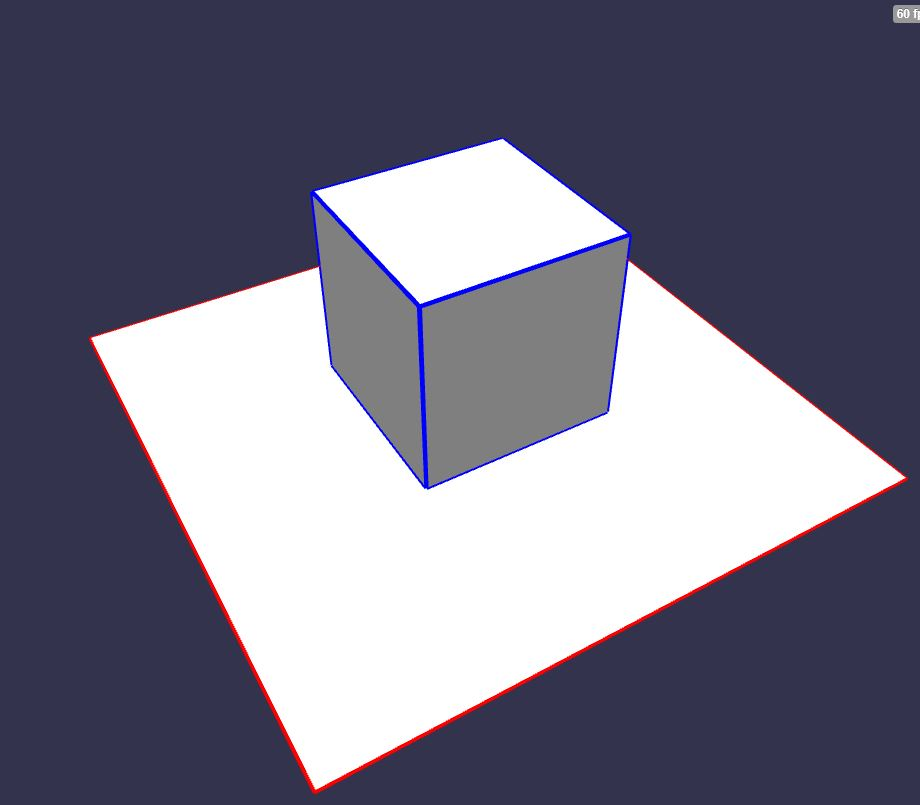# Rendering Edges

BABYLON.EdgesRenderer is a tool used to render edges on top of a mesh. Edges are rendered between two faces if the dot product of their normals is less than epsilon.You can enable edges rendering like this:

const box = BABYLON.MeshBuilder.CreateBox("box1", { size: 2 }, scene);box.enableEdgesRendering();box.edgesWidth = 4.0;box.edgesColor = new BABYLON.Color4(0, 0, 1, 1);

The enableEdgesRendering can be called with a custom epsilon (default value is 0.95).

box.enableEdgesRendering(0.9999);

Dot product is the cosine of the angle between the vectors, so for default epsilon 0.95 the angle is acos(.95) ~= 18 degrees - so if the angle between two faces is less than that no line gets drawn.

If you need to turn-off edges rendering:

box.disableEdgesRendering();

You can try edges rendering here: Edge Rendering Example 1

If your mesh has instances, you can either use a renderer for each instance by enabling the edges rendering for each instance as explained above, or by using the source mesh renderer for all instances.

To do this, just set:

sourceMesh.edgesShareWithInstances = true;

PG: Edge Rendering Example 2